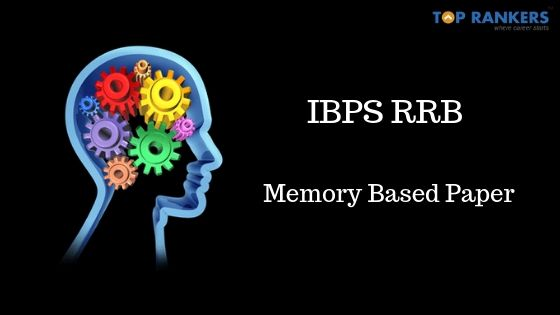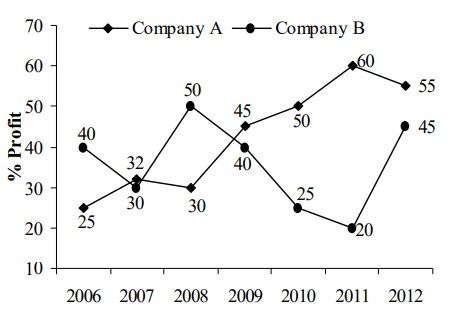# IBPS RRB Clerk Memory Based Paper 2019Institute of Banking Personnel Selection is conducting the Officer Scale I and Office Assistant Exam in the month of August 2019. To help all the candidates who are appearing for IBPS RRB Exam we are providing IBPS RRB Memory Based Paper for cracking Office Assistant Exam and Officer Scale I.

• In this post, we have provided questions that are asked in previous year IBPS RRB Clerk examinations.
• Go through the detailed IBPS RRB Exam Analysis for Clerk post that is conducted on August 17, 18, and 25, 2019.
• Generally, Memory Based Question Papers consists of the previous year examination questions that can help students to know what type of questions can appear in the exam.
• It is said by experts that most of the questions repeat from IBPS RRB Previous Year Question Papers with slight changes in the numerical.

## IBPS RRB Memory Based Paper 2019

### IBPS RRB Office Assistant Memory Based Paper

Here is a list of IBPS RRB Clerk Exam questions to enhance candidate’s preparation for IBPS RRB Clerk exam 2019. Go through the detailed IBPS RRB Clerk Memory Based Paper here to know which questions appeared in IBPS RRB Today’s clerk exam.

We have provided section-wise IBPS RRB Office Assistant Questions that includes various questions from previous year exam.

### IBPS RRB Clerk Memory Based Questions for Quantitative Aptitude

Q. Find the wrong number in the series: 1, 2, 5, 16, 65, 328

Ans: 328

Q. Find the wrong number in the series: 4, 11, 25, 46, 74, 129

Ans: 129

Q. Find the wrong number in the series: 84, 96, 83, 95, 80, 94, 81

Ans: 82

Q. Find the wrong number in the series: 3, 5, 8, 17, 33, 58, 94

Ans: 5

Q. How many days will there be from 26th January 2004, to 15th May 2004?

A.  110
B.  111
C.  112
D.  113

Q. The year next to 1990 will have the same calendar as that of the year 1990?

A. 1995
B. 1997
C. 1996
D. 2001

Q. A light was seen at intervals of 13 seconds. It was seen for the first time at 1 hr. 54 min 50 secs. a.m. and the last time at 3 hrs. 17 min. 49 secs. a.m. How many times was the light seen?

(A) 360

(B) 375

(C) 378

(D) 384

Q.  David got two and a half time as many marks in English as in History. If his total marks in the two subjects are 140, the marks obtained by him in English are:

(A) 40

(B) 75

(C) 90

(D) 100

Q. The total number of digits used in numbering the pages of a book having 366 pages, is:

(A) 732

(B) 990

(C) 1098

(D) 1305

### IBPS RRB Clerk Memory Based Questions for Reasoning

Q. Find the number of pairs possible from ATTENTION word

Q. Which letter is seventh from the left of 16th from right in the English alphabet?

(A) D

(B) W

(C) R

(D) E

Q. If the English alphabet is written in reverse order then- Which letter is 5th at right of 12 from right?

(A) Q

(B) P

(C) G

(D) R

Q.  If the English alphabet is written in reverse order then- If the 2nd half of an English alphabet is written in reverse order, which letter will be the 5th at left from 21st letter from left?

(A) L

(B) X

(C) M

(D) Q

Q. Arrange the following in the order of the dictionary.

1. Scenery 2. Science 3. Scandal 4. School 5. Scatter

Q.  A word is given in each of the following questions. Then four options are given below each word. One of the options is a word which cannot be made using the letters of the given word. Identify that option.

RECOMMENDATION:

(A) NATION

(B) COMMENT

(C) COMMAN

(D) RECOMMENDS

Q. A word is given in each of the following questions. Then four options are given below each word. One of the options is a word which cannot be made using the letters of the given word. Identify that option.

LEDMENTNOWGEACK:

(A) KNOWLEDGE

(B) GENTLE

(C) AGENCY

(D) LODGE

### IBPS RRB Officer Scale I Memory Based Paper

This post is to enhance candidate’s preparation by providing them with Previous year IBPS RRB Officer Scale I Exam Questions. These questions are compiled and prepared by a panel of experts and say that preparing from previous year questions will help in solving most of the questions.

Go through the section-wise Important IBPS RRB Memory Based Paper from Previous Year Exam and enhance your revision. These are just a few important Memory Based Paper Questions, but candidates need to refer to the previous year papers of IBPS RRB Exam.

### IBPS RRB Memory Based Questions for Quantitative Aptitude

Q1.  What approximate value of the equation:  423.62 – 269.89 ÷ (11.9% of 74.98) = ?

A. 525

B. 455

C. 395

D. 650

E. 275

Q2. find the missing term:  8, 9, 15, 32, ?, 250.5
A. 82.5

B. 47.5

C. 62.5

D. 37.5

E. 64.5

Q3. Approximately what is the percentage of candidates qualified over appeared from all the institutes together in 2017?

A. 98%

B. 75%

C. 64%

D. 86%

E. 69%

Q4. A bag contains 6 red, 7 blue, and 8 green balls. Three balls are drawn randomly. What is the probability that the balls are drawn contain exactly two blue balls?

A.  147/665

B. 518/665

C. 54/455

D. 44/455

E. 401/455

Q5. Two pipes can separately fill a tank in 10 hours and 40 hours respectively. Both the pipes are opened together to fill the tank, but when the tank is half-filled a leakage develops, through which 1/2 of the water supplied by both the pipes leaks out. What is the total time taken to fill the tank?

A. 8 hours

B. 10 hours

C. 12 hours

D. 16 hours

E. 18 hours

Q6. A square park is surrounded on the outside by a path 2m wide. If the area of the path is 184 sq m, then what will be the area of the park?

A. 400 sq m

B. 441 sq m

C. 484 sq m

D. 529 sq m

E. 576 sq m

Q7. Find the value of (1884% of 73) ÷ 25.05 = ?

A. 35

B. 45

C. 55

D. 65

E. 75

Q8. The sum of the ages of A, B, and C at present is 85 years. 10 years ago the ratio of their ages was 1 : 3: 7. The present age of C is what percentage of the present age of B?

A. 55.55%

B. 80%

C. 120%

D. 180%

E. 300%

The following graph shows the net percentage profit of two companies, A and B for the period 2006 to 2012Q9. If the income of Company A in the year 2007 is Rs 85.8 lakh, then what will be its expenditure (in Rs) in that year?

A. 56 lakh

B. 65 lakh

C. 72.8 lakh

D. 97.64 lakh

E. 113.256 lakh

Q10. If in the year 2012 the expenditure of Company A was Rs 90.6 lakh, what was its income (in Rs) in that year?

A.  139.18 lakh

B. 148 lakh

C. 138.2 lakh

D. 140.43 lakh

E. 144.64 lakh

Q11. In which of the following years is the percentage increase in the profit of Company A the highest over the preceding year?

A. 2007

B. 2009

C. 20010

D. 2011

E. None of these

Q12. In which of the following years is the difference between the income and the expenditure of Company B the maximum?

A. 2006

B. 2008

C. 20011

D. 2012

E. None of these

### IBPS RRB Memory Based Questions for Reasoning

Q(1-5) Study the information given below and answer the given questions:
In a certain code ‘facing problems with health’ is coded as ‘mlp hlt ngi snk’, ‘health problems on rise’ is coded as ‘hlt sa rtv mlp’, ‘rise with  very challenge’ is coded as ‘snk rtv lne riy’ and ‘facing challenge each day’ is coded as ‘ngi riy nop hus’.

Q1. What could be a code for “lne”?

A. facing

B. with

C. every

D. rise

E. challenge

Q2. “riy rtv roi” could be a code for which of the following?

A. rise above challenge

B. rise health challenge

C. day rise challenge

D. with rise challenge

E. challenge every rise

Q3. Which of the following is the code for “facing”?

A. nop

B. rtv

C. ngi

D. snk

E. sa

Q4. “riy snk mlp” could be a code for which of the following?

A. problem every day

B. challenge with health

C.  with health day

D. every challenge facing

E. challenge facing with

Q5. Which of the following is the code for ‘day’?

A. riy

B. nop

C. ngi

D. hus

E. Cannot be determined

Q(6-11) Study the information given below and answer the given questions.
Eight friends A, B, C, D, E, F, G, and H are sitting in a circle, but not necessarily in the same order. Four of them are facing outside and four of them are facing the center.

• E faces outside. Both the immediate neighbors of E face the center. H sits second to the right of E. B sits third to the left of E
• D faces the center. Both the immediate neighbors of D face outside.
• G sits second to the left of A. B sits third to the right of H
• F is an immediate neighbor of D. C is an immediate neighbor of G.
• D is not an immediate neighbor of B.

Q6. Who amongst the following sits on the immediate right of H?

A. A

B. D

C. C

D. G

E. None of these

Q7. Who amongst the following sits third to the right of A?

A. D

B. E

C. F

D. A

E. None of these

Q8. Four of the following five are alike in a certain way, based on the information given above and so form a group. Which is the one that does not belong to that group?

A. HA

B. FH

C. GC

D. DA

E. AE

Q9. If all the people are made to sit in alphabetical order, in a clockwise direction, starting from A, the position of whom amongst the following remains the same (excluding A)?

A. E

B. F

C. C

D. G

E. None of these

Q10. How many people are seated between A and C (counting clockwise from A)?

A. Two

B. Four

C. None

D. One

E. Three

Q11. Who amongst the following sits exactly between F and C (and is also their neighbor)?

A. E

B. B

C. G

D. A

E. None of these